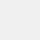# Math Games, Learn Add Multiply

Version 17.3
 Install +50 K From 332 Rates 4.6 Category Kids Size 15 MB Last Update 2023 July 13# Math Games, Learn Add Multiply

GunjanApps Studios
Version 17.3
 Install +50 K From 332 Rates 4.6 Category Kids Size 15 MB Last Update 2023 July 13
View the antivirus scan results

## Introduction

★★★ Math App on Google Play ★★★

Math Games Languages: English, Spanish, German, Italian, Portuguese, French, Russian, Indonesian, Malay, Vietnamese, Japanese, Korean, Hindi, Bengali & Ukrainian

Math Games, Learn Add, Subtract, Multiply & Divide Features :
- Subtraction games: Subtracting numbers to solve the equations
- Multiplication games: Multiplication tables learning and duel play mode
- Division games: Practice and learn Division tables in new Math app
- Exponential and square root: counting math puzzles for kids and adults
- Fractions: Basic arithmetic to fraction addition, subtraction & multiplication for kids and adults
- Dual Mode: Split-screen interface for two players with endless math worksheets
- Multiply number game: children learn math skills and multiplication tables
- Cool Maths games, math puzzles, brain teasers, and brain math puzzles all in one
- Maths Times Tables along with Fun adding, subtracting, multiplying and dividing
- Math classic 15 Puzzle, Sudoku and more math exercises for brain
- NEW Online Multiplayer math game

Each category has different play modes to improve math skills - Play, Learn, Quiz, Practice, Duel and Test. Math games for kids can be Educational Learning for kids or brain training app for Adults. Basic and simple Math Game of addition, subtraction, multiplication and division with colorful worksheets. Each set of worksheet shows a score after completion.

Mathematical calculations to play and practice with simple addition, subtraction, multiplication and division. Now download and play Math games for free on android ! Improve your mathematics skills or learn counting numbers. The games are so simple and easy even the youngest kids can play it. Math Games - Addition, Subtraction, Multiplication & Division, can name it xtra math as well.

Fun addition and subtraction games with multiplication tables, designed for all smartphones and tablets, no internet connection needed. Including Times Tables Multiplications and Division. With this educational app, parents, teachers and educators can help children to learn faster. This Math Genius game is a fun game designed mainly around the topics of addition, subtraction, multiplication and division. Cool math games to play & challenge your friend and have xtra math fun. We keep on adding new math game for kids in 1st grade, 2nd grade, 3rd grade, 4th grade, and 5th grade.

User Reviews - 332 Rates
4.6 from 5
5
4
3
2
1سایید
2023/09/04
تو عمرم همچین برنامه کاملی ندیده بودم واقعا از سازنده ممنونموحید
2023/07/31
عالیمهسا شریفی
2023/08/02
عالیییی